# Construct CUSUM charts for hospital performance

## Overview

This vignette describes CUSUM charts based on a simulated false-signal-probability for hospital performance data in the R package cusum. This is a practical guide to constructing and evaluating non-risk-adjusted and risk-adjusted CUSUM charts following Steiner et al. (Biostatistics 1.4 (2000), pp. 441-52).

The cusum packages takes different factors into account that influence the signal rate of CUSUM charts. Some are given by the process to be monitored; these factors are:

• number of patients: How many observations do we expect in a monitoring period (e.g. a month/a year)?
• risk-adjustment: Is risk adjustment available? Can we allocate different risks to different observations?
• if yes:
• patient risks: What are these risks and how are they distributed?
• if no:
• accepted failure probability: What kind of failure rate do we expect on average?

The primary control input when constructing a CUSUM chart is the control limit. The control limit signals performance deterioration once crossed by the cumulated sum.

The control limit depends on a number of variables:

• the desired target odds multiplier associated to an out-of-control process
• the false signal probability accepted
• number of simulations

## Motivating example

To illustrate how cusum can be used for monitoring, we employ a simple and artificial data set generated to closely follow the performance data of German hospitals for one non-risk-adjusted performance indicator and one risk-adjusted performance indicator in 2016 and 2017.

risk-adj. Indicator Description Further explanation (in German)
NO Ratio of observed to expected cases of severe stroke or death under open carotid stenosis surgery pdf (p4)
YES Preoperative stay more than 24 hours for patients with proximal femur fracture pdf (p23)

data("cusum_example_data", package = "cusum")

#>   t     y year
#> 1 1 FALSE 2016
#> 2 2 FALSE 2016
#> 3 3 FALSE 2016
#> 4 4 FALSE 2016
#> 5 5 FALSE 2016
#> 6 6 FALSE 2016

data("racusum_example_data", package = "cusum")

#>   t     y   score year
#> 1 1 FALSE 0.00237 2016
#> 2 2 FALSE 0.00237 2016
#> 3 3 FALSE 0.02412 2016
#> 4 4 FALSE 0.01893 2016
#> 5 5 FALSE 0.00725 2016
#> 6 6 FALSE 0.00810 2016

First, CUSUM charts are constructed on performance data from 2016 (Phase I), and then applied and evaluated on performance data from 2017 (Phase II).

cusum_example_p1 <- cusum_example_data[cusum_example_data$year == 2016, ] cusum_example_p2 <- cusum_example_data[cusum_example_data$year == 2017, ]

racusum_example_p1 <- racusum_example_data[racusum_example_data$year == 2016, ] racusum_example_p2 <- racusum_example_data[racusum_example_data$year == 2017, ]

### Simulation of CUSUM Control Limits

We get the control limit of our CUSUM chart by simulating for a false signal probability depending on sample size and accepted failure probability.

We can estimate the accepted failure probability by taking the average of Phase I. Alternatively, we could also define an accepted failure probability politically

failure_probability <- mean(cusum_example_p1$y) n_patients <- nrow(cusum_example_p1) Then, control limits can be simulated using cusum_limit_sim.  cusum_limit <- cusum_limit_sim(failure_probability, n_patients, odds_multiplier = 2, n_simulation = 1000, alpha = 0.05, seed = 2046) print(cusum_limit) #>  6.498476 ### Applying CUSUM Charts Monitoring via CUSUM charts is applied on performance data from 2017 (Phase II) and the control limit cusum_limit. It can be calculated using cusum. patient_outcomes <- cusum_example_p2$y

cusum_cs <- cusum(failure_probability,
patient_outcomes,
limit = cusum_limit,
odds_multiplier = 2,
reset = FALSE)

#>   t failure_probability ct signal    limit
#> 1 1               0.216  0      0 6.498476
#> 2 2               0.216  0      0 6.498476
#> 3 3               0.216  0      0 6.498476
#> 4 4               0.216  0      0 6.498476
#> 5 5               0.216  0      0 6.498476
#> 6 6               0.216  0      0 6.498476
plot(cusum_cs)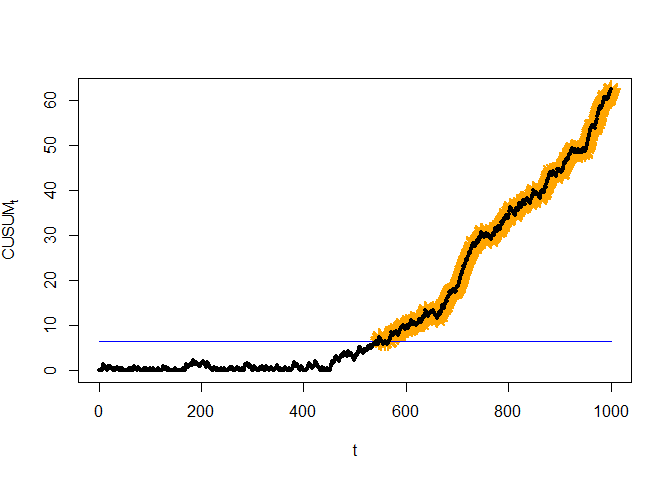Performance is as expected during the first half of monitoring, and then deteriorates. We get a signal at t=547. If reset==TRUE, the CUSUM resets after each signal.

cusum_cs <- cusum(failure_probability,
patient_outcomes,
limit = cusum_limit,
odds_multiplier = 2,
reset = TRUE)

plot(cusum_cs)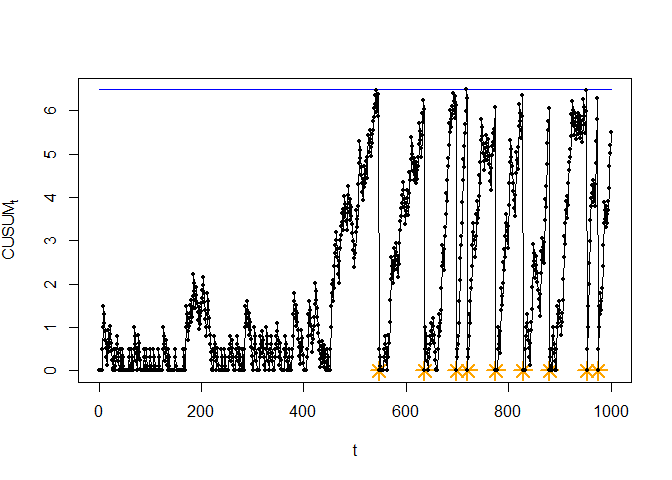### Evaluating CUSUM charts

The false signal probability of a CUSUM chart can be simulated using cusum_alpha_sim given a predefined control limit.

n_patients <- nrow(cusum_example_p2)

cusum_alpha <- cusum_alpha_sim(failure_probability,
n_patients,
odds_multiplier = 2,
n_simulation = 1000,
limit = cusum_limit,
seed = 2046)

print(cusum_alpha)
#>  0.05

We see that cusum_alpha equals our previously defined false signal probability of 0.05.

### Simulation of RA-CUSUM Control Limits

Control limits of RA-CUSUM charts are simulated for a false signal probability depending on sample size and risk distribution.

RA-CUSUM Control limits can be simulated using racusum_limit_alpha.



patient_outcomes <- racusum_example_p2$y racusum_cs <- racusum(patient_risks, patient_outcomes, limit = racusum_limit, odds_multiplier = 2, reset = FALSE) plot(racusum_cs)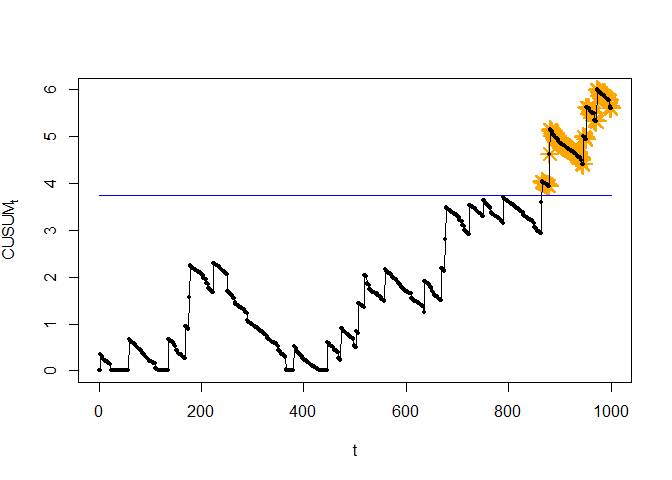Performance is as expected during the first half of monitoring, and then deteriorates. We get a signal at t=865. If reset==TRUE, the CUSUM resets after each signal.  racusum_cs <- racusum(patient_risks, patient_outcomes, limit = racusum_limit, odds_multiplier = 2, reset = TRUE) plot(racusum_cs)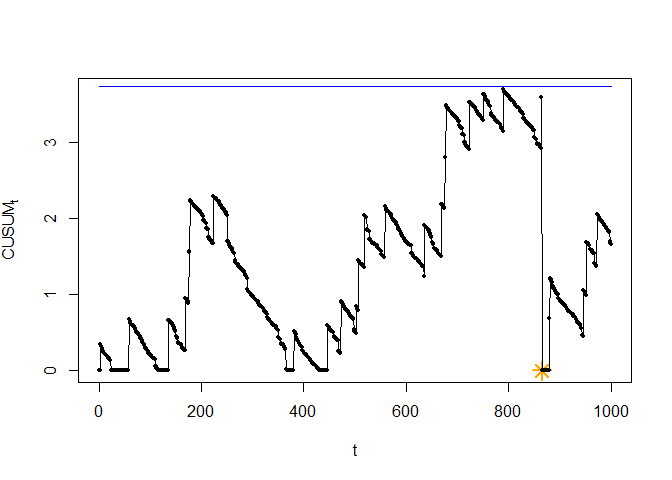### Evaluating RA-CUSUM charts The false signal probability of a CUSUM chart can be simulated using cusum_alpha_sim. racusum_alpha <- racusum_alpha_sim(patient_risks, odds_multiplier = 2, n_simulation = 1000, limit = racusum_limit, seed = 2046) print(racusum_alpha) #>  0.058 We see that racusum_alpha is similar to our previously defined false signal probability of 0.05. Deviation is possible due to a slight change in risk population. ## CUSUM Chart for process improvement CUSUM charts for detecting process improvements can be constructed similarly, but the CUSUM statistic is restricted to non-positive values.  cusum_limit_improve <- cusum_limit_sim(failure_probability, n_patients, odds_multiplier = .5, n_simulation = 1000, alpha = 0.5,seed = 2046) cusum_cs_improve <- cusum(failure_probability, patient_outcomes = cusum_example_p2$y,
limit = cusum_limit_improve,
odds_multiplier = .5)

plot(cusum_cs_improve)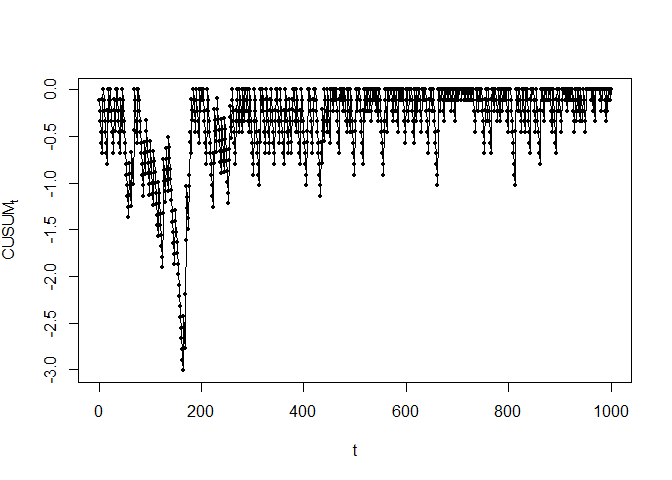cusum_alpha_sim(failure_probability,
n_patients,
odds_multiplier = 0.5,
n_simulation = 1000,
limit = cusum_limit_improve,
seed = 2046)
#>  0.505